# Measuring Strain with Surface Acoustic Wave Devices

## Non-Contact Strain Measurement Utilizing Rayleigh Waves

### Surface Acoustic Waves (SAW)—Rayleigh Waves

Lord Rayleigh, an English scientist, had hypothetically demonstrated3 in 1885 that waves could be propagated across the plane boundary between a vacuum (or an adequately rarefied medium, for example, air) and a linearly elastic half-space, where the waves’ amplitude decays exponentially with depth. It was predicted by Rayleigh that such waves can be a significant component of earthquakes, a fact to be established years later in the 1920s because of the introduction of seismographic recordings.

About 45 years later, such waves4 were generated by White and Voltmer from the University of California. These waves are often known as Rayleigh Waves or surface acoustic waves (SAW), on the free surface of an elastic and isotropic substrate, such as quartz.

As shown in Figure 1, a pattern of displacements is produced by the action of the wave on the solid, and the dots in these displacements indicate material particles, which are nominally equidistant horizontally as well as vertically in the absence of wave motion.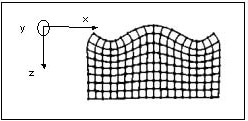Figure 1. Surface acoustic waves

Equations (1) and (2) give the displacement components along the x and z axes, respectively.5

 (1)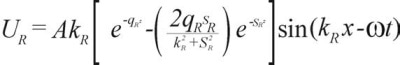(2)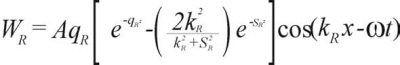Where:

• A denotes an amplitude constant
• kR stands for the Rayleigh wave number
• kT = ω(ρ/μ)1/2 are the wave numbers for transverse modes
• kL = ω(ρ/(λ + μ))1/2 are the wave numbers for longitudinal modes
• μ and λ are the elastic Lame constants, ω is the circular frequency, and ρ is the material density
• sR = (kRkT)1//2 and qR = (kRkL)1/2
• x is the in-plane displacement, t is the time, and z is the vertical displacement

Since UR and WR — the components of displacement in the Rayleigh wave along the x and z axes, respectively — are moved in phase by π/2, the oscillation mode of particles supporting a Rayleigh wave is a retrograde ellipse, the normal (vertical) displacement of which reaches its highest test amplitude at a depth of about 0.2 λR (where λR refers to the Rayleigh wavelength) and subsequently decays to zero within a couple of wavelengths from the surface. In addition, it contains a displacement component in the plane of the solid surface.

Given that the material characteristics of the surface layer of a sample have an effect on the attenuation and velocity of Rayleigh waves, the former can be used to assess the remaining stresses in the surface layer, and also the mechanical and thermal characteristics of the sample’s surface layer. The application of Rayleigh waves is based on this phenomenon for the non-intrusive surface testing of components, and in particular, strain measurement. A transducer design that can measure such phenomena is the requirement.

## Surface Acoustic Wave Transducer Construction

A surface acoustic wave has an important characteristic — that is, its phase velocity is slightly smaller when compared to the phase velocity of a bulk wave in that direction. Additionally, when compared to the velocity of electromagnetic radiation in vacuo, the Rayleigh wave velocity is about 105 times slower. Therefore, for the same frequency, the elastic wave’s wavelength is less than the corresponding electromagnetic wave’s wavelength by a factor of 105 for the same frequency.

This outcome has an instant significance on the geometry of the ensuing gauge and represents the philosophy behind surface wave technology. The devices themselves can be relatively smaller than their electromagnetic equivalents, with the added benefit that the surface wave can be immediately perceived anywhere along its path. Significantly, it can be demonstrated3 that phase velocity dispersion is not exhibited by the Rayleigh wave; in other words, the velocity does not depend on frequency.

As shown in Figure 2, metallic film transducers on the surface of a piezoelectric material produce the Rayleigh waves. These metallic film transducers possess an interdigital configuration, which can be immediately developed through classic integrated circuit technology.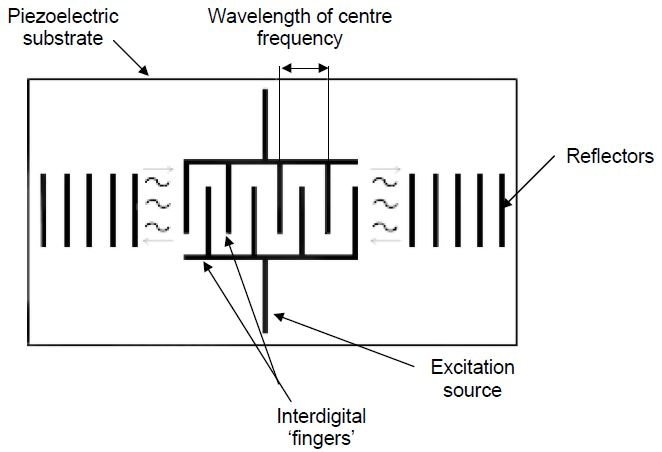Figure 2. Outline of a basic SAW transducer

The period of the wave produced is determined by the period of the fingers. When an alternating voltage is applied to the transducer, the surface of the piezoelectric material undulates intermittently, thereby creating waves. Reflected by an analogous array, these waves create a one-port structure, the impedance of which can be set at a specified frequency, usually 50 Ω.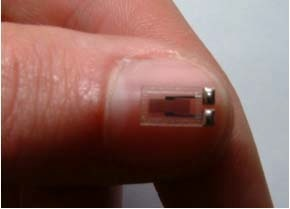Figure 3. 200 MHz SAW resonator made of quartz shown against thumbnail

The choice of an appropriate piezoelectric substrate governs the operation of a SAW transducer for the measurement of strain. This piezoelectric substrate can be connected to the material to be stressed. A strain induced by the stress can be either in a state of compression or in a state of tension. The transducer’s sensitive axis is longitudinal in the direction of wave propagation. The spacing of the inter-digital electrodes will be changed by strain change, and therefore, this is the reason for the operating frequency.

For instance, for a 500 MHz excitation frequency, 1000 με of tension will reduce the frequency by 500 KHz (simply: 1000.e-6.500.e6); on the other hand, a compressive strain will raise the frequency by the same amount. The transducer’s quality factor (Q) is high, usually 104. Therefore, the impedance can be measured to control a tracking oscillator; this can indicate the resonant frequency within a few Hertz.

From the method described, it is obvious that the output signal will be in the frequency domain as illustrated in Figure 4.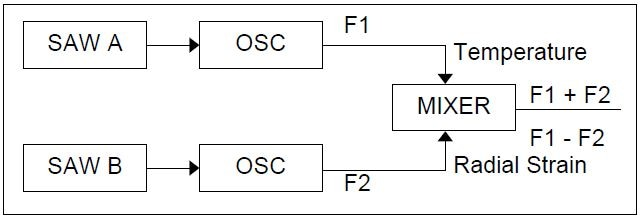Figure 4. Schematic of frequency domain SAW signals

From an application standpoint, this has a number of benefits, especially in variable speed electrical machines where drive electronic generated noise can easily contaminate low-frequency signals. Designed at the 50 Ω standard, the SAW impedance means there is normally less noise than in its traditional resistance gauge equivalent (resistance is often 100–350 Ω). The highest power of the SAW signal is in the region of 25 mW, and therefore, when compared to, for example, a 10 V half bridge resistive strain gauge system in which the overall power of the output strain signal is in the region of 1 μW, the SAW provides a more powerful solution for the measurement of strain. Although piezoelectric transducers are available for dynamic strain sensing, they deliver poor temperature performance and have a high output impedance, both of which are major drawbacks.

## Application of SAW Devices for Measuring Strain

### Torque Measurement

One common device utilized by development engineers is torque (radial strain) transducers. A better understanding of rotational speed and torque can be utilized to indicate power, from which electrical machines, transmissions, the efficiency of gearboxes, and many other systems can be quickly assessed. In order to apply the SAW element principle, a couple of devices — one positioned to observe the principal tensile strain and the other positioned so as to be responsive to the principal compressive strain — are utilized in a half bridge, similar to the configuration of standard resistive strain gauging.

It must be noted that in the absence of axial forces and bending moments, the principal stress planes lie vertical to each other at 45° to the plane about which the tensional moment is induced. This is shown in Figure 5.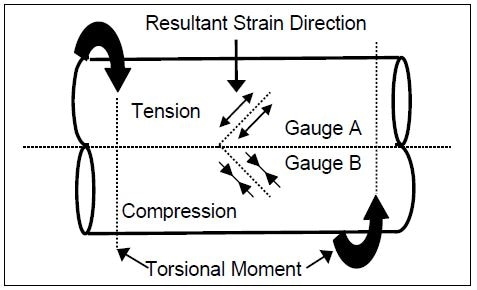Figure 5. SAW gauge arrangement for torque measurement

The SAW produces two frequencies that are combined together to create the difference and /or sum signals. This difference signal is a measurement of induced strain owing to the twisting moment, and therefore, based on the understanding of the governing equations and the material properties, the torque is implied. The sum signal, on the other hand, is a measure of shaft temperature.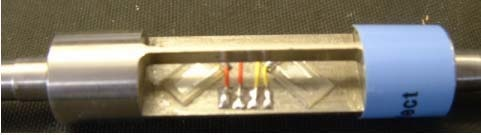Figure 6. A typical structure — shaft with two SAWs attached

An electromagnetic coupling device is used to realize the coupling of the signals to and from the machine shaft. This electromagnetic coupling device contains a pair of concentric loops, detached by an appropriate distance; one concentric loop is fixed to the shaft housing, while the other is attached to the rotating shaft offering non-contact interrogation, which is intrinsically safe. Figure 7 illustrates this coupling.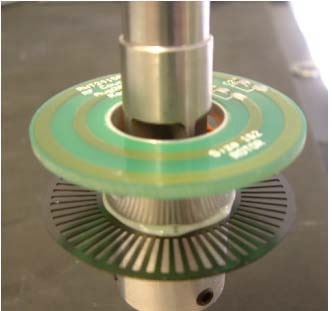Figure 7. Electromagnetic coupling device mounted onto the shaft

The main frequency of oscillation can be selected to lie between 100 and 1000 MHz with the difference frequency changing up to 1 MHz. A transducer like that has the following specification:

• Bandwidth: >1 MHz
• Linearity: 0.1%
• Resolution: 1 part in 106

## Conclusions

This article has shown how SAW devices can be utilized as strain gauges functioning in the frequency domain. A better sensitivity over previous devices by two orders of magnitude is not impractical. Moreover, the low power needs, combined with low impedance, make these devices better than traditional resistance gauges. Thanks to their ease of manufacture and simplicity, it is likely that these devices will work well into the 21st century, just like the resistance gauge that has served at the time of the 20th century.

## References

1. A Lonsdale & B Lonsdale EU Patent EP518900, USA Patent 5585571
2. “Theory Of Elasticity,” Timoshenko, S. P., & Goodier, J. N., Third Ed., 1970, McGraw-Hill Inc.
3. Lord Rayleigh (J. Strutt): “On waves propagated along the plane surface of an elastic solid,” Proc. Lon. Math. Soc., (17): 4-11, 1885.
4. White, R.M. and Voltmer, F.W.: “Direct piezoelectric coupling to surface elastic waves,” Applied Physics. Letters, (7): pp 314-316, 1965.
5. Viktorov, I.V.: “Rayleigh and Lamb Waves—Physical theory and applications,” Plenum Press, New York, 1967.This information has been sourced, reviewed and adapted from materials provided by Sensor Technology.

## Citations

• APA

Sensor Technology. (2019, June 07). Measuring Strain with Surface Acoustic Wave Devices. AZoSensors. Retrieved on April 02, 2020 from https://www.azosensors.com/article.aspx?ArticleID=1640.

• MLA

Sensor Technology. "Measuring Strain with Surface Acoustic Wave Devices". AZoSensors. 02 April 2020. <https://www.azosensors.com/article.aspx?ArticleID=1640>.

• Chicago

Sensor Technology. "Measuring Strain with Surface Acoustic Wave Devices". AZoSensors. https://www.azosensors.com/article.aspx?ArticleID=1640. (accessed April 02, 2020).

• Harvard

Sensor Technology. 2019. Measuring Strain with Surface Acoustic Wave Devices. AZoSensors, viewed 02 April 2020, https://www.azosensors.com/article.aspx?ArticleID=1640.

## Tell Us What You Think